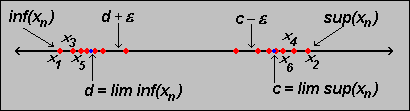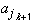#Interactive Real Analysis

Next | Previous | Glossary | Map

## 3.4. Lim Sup and Lim Inf

### Proposition 3.4.4: Characterizing lim sup and lim inf

Letbe an arbitrary sequence, and let c = lim sup aj and d = lim inf aj. Then
1. there is a subsequence converging to c
2. there is a subsequence converging to d
3. dlim inflim supc for any subsequence {}
If c and d are both finite, then: given any> 0 there are arbitrary large j such that aj > c -and arbitrary large k such that ak < d +.### Proof:

First let's assume that c = lim sup{aj} is finite, which implies that the sequence {aj} is bounded. Recall the properties of the sup (and inf) for sequences:
If a sequence is bounded above, then given any> 0 there exists at least one integer k such that ak > c -Now take any> 0. Then
Ak = sup{ak, ak+1, ...}
so by the above property there exists an integer jk > k such that
Ak >> Ak -/ 2
or equivalently
| Ak -| </ 2
We also have by definition that Ak converges to c so that there exists an integer N such that
| Ak - c | </ 2
But now the subsequence {} is the desired one, because:
|- c | = |- Ak + Ak - c ||- Ak | + | Ak - c |
</ 2 +/ 2 =if jk > N. Hence, this particular subsequence of {an} converges to c.

The proof to find a subsequence converging to the lim inf is similar and is left as an exercise.

Statement (3) is pretty simple to prove: For any sequence we always have that

inf{ak, ak+1, ... }sup{ak, ak+1, ... }
Taking limits on both sides gives lim inf(an)lim sup(an) for any sequence, so it is true in particular for any subsequence.

Next take any subsequence of {an}. Then:

inf(ak, ak+1, ...)inf(,, ...)
because an infimum over more numbers (on the left side) is less than or equal to an infimum over fewer numbers (on the right side). But then
dlim inf()
The proof of the inequality lim sup()c is similar. Taking all pieces together we have shown that
dlim inflim supc
for any subsequence {}, as we set out to do.

It remains to show that given any> 0 there are arbitrary large j such that aj > c -(as well as the corresponding statement for the lim inf d).

But previously we have found a subsequence {} that converges to c so that there exists an integer N such that

|- c | <if k > N. But that means that -<- c <which implies that
c -<< c +as long as k > N. But that of course means that there are arbitrarily large indices - namely those jk for which k > N - with the property that> c -as required. Hence, we have shown the last statement involving the lim sup, and a similar proof would work for the lim inf.

All our proofs rely on the fact that the lim sup and lim inf are bounded. It is not hard to adjust them for unbounded values, but we will leave the details as an exercise.